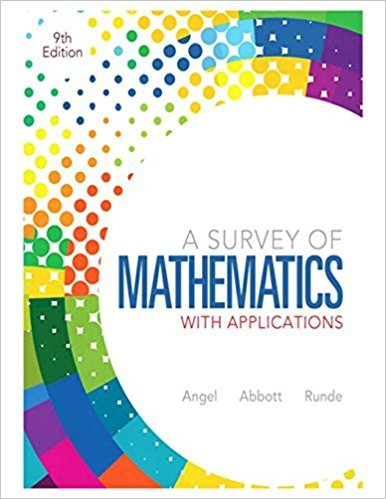×
×

# Solutions for Chapter 1.1: Critical Thinking Skills## Full solutions for A Survey of Mathematics with Applications | 9th Edition

ISBN: 9780321759665Solutions for Chapter 1.1: Critical Thinking Skills

Solutions for Chapter 1.1
4 5 0 255 Reviews
18
1
##### ISBN: 9780321759665

Since 55 problems in chapter 1.1: Critical Thinking Skills have been answered, more than 74083 students have viewed full step-by-step solutions from this chapter. A Survey of Mathematics with Applications was written by and is associated to the ISBN: 9780321759665. This expansive textbook survival guide covers the following chapters and their solutions. Chapter 1.1: Critical Thinking Skills includes 55 full step-by-step solutions. This textbook survival guide was created for the textbook: A Survey of Mathematics with Applications, edition: 9.

Key Math Terms and definitions covered in this textbook
• Back substitution.

Upper triangular systems are solved in reverse order Xn to Xl.

• Circulant matrix C.

Constant diagonals wrap around as in cyclic shift S. Every circulant is Col + CIS + ... + Cn_lSn - l . Cx = convolution c * x. Eigenvectors in F.

• Cofactor Cij.

Remove row i and column j; multiply the determinant by (-I)i + j •

• Companion matrix.

Put CI, ... ,Cn in row n and put n - 1 ones just above the main diagonal. Then det(A - AI) = ±(CI + c2A + C3A 2 + .•. + cnA n-l - An).

• Covariance matrix:E.

When random variables Xi have mean = average value = 0, their covariances "'£ ij are the averages of XiX j. With means Xi, the matrix :E = mean of (x - x) (x - x) T is positive (semi)definite; :E is diagonal if the Xi are independent.

• Dot product = Inner product x T y = XI Y 1 + ... + Xn Yn.

Complex dot product is x T Y . Perpendicular vectors have x T y = O. (AB)ij = (row i of A)T(column j of B).

• Full row rank r = m.

Independent rows, at least one solution to Ax = b, column space is all of Rm. Full rank means full column rank or full row rank.

• Hessenberg matrix H.

Triangular matrix with one extra nonzero adjacent diagonal.

• Identity matrix I (or In).

Diagonal entries = 1, off-diagonal entries = 0.

• lA-II = l/lAI and IATI = IAI.

The big formula for det(A) has a sum of n! terms, the cofactor formula uses determinants of size n - 1, volume of box = I det( A) I.

• Left inverse A+.

If A has full column rank n, then A+ = (AT A)-I AT has A+ A = In.

• Linear transformation T.

Each vector V in the input space transforms to T (v) in the output space, and linearity requires T(cv + dw) = c T(v) + d T(w). Examples: Matrix multiplication A v, differentiation and integration in function space.

• Minimal polynomial of A.

The lowest degree polynomial with meA) = zero matrix. This is peA) = det(A - AI) if no eigenvalues are repeated; always meA) divides peA).

• Norm

IIA II. The ".e 2 norm" of A is the maximum ratio II Ax II/l1x II = O"max· Then II Ax II < IIAllllxll and IIABII < IIAIIIIBII and IIA + BII < IIAII + IIBII. Frobenius norm IIAII} = L La~. The.e 1 and.e oo norms are largest column and row sums of laij I.

• Projection matrix P onto subspace S.

Projection p = P b is the closest point to b in S, error e = b - Pb is perpendicularto S. p 2 = P = pT, eigenvalues are 1 or 0, eigenvectors are in S or S...L. If columns of A = basis for S then P = A (AT A) -1 AT.

• Rank one matrix A = uvT f=. O.

Column and row spaces = lines cu and cv.

• Right inverse A+.

If A has full row rank m, then A+ = AT(AAT)-l has AA+ = 1m.

• Spectral Theorem A = QAQT.

Real symmetric A has real A'S and orthonormal q's.

• Tridiagonal matrix T: tij = 0 if Ii - j I > 1.

T- 1 has rank 1 above and below diagonal.

• Volume of box.

The rows (or the columns) of A generate a box with volume I det(A) I.

×